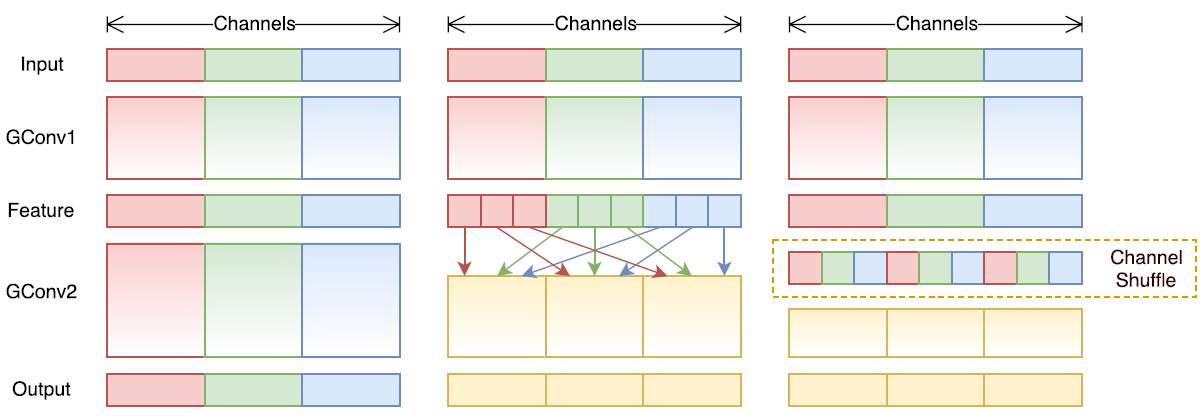## 引言

ShuffleNet是旷视科技最近提出的一种计算高效的CNN模型，其和MobileNet和SqueezeNet等一样主要是想应用在移动端。所以，ShuffleNet的设计目标也是如何利用有限的计算资源来达到最好的模型精度，这需要很好地在速度和精度之间做平衡。ShuffleNet的核心是采用了两种操作：pointwise group convolution和channel shuffle，这在保持精度的同时大大降低了模型的计算量。目前移动端CNN模型主要设计思路主要是两个方面：模型结构设计和模型压缩。ShuffleNet和MobileNet一样属于前者，都是通过设计更高效的网络结构来实现模型变小和变快，而不是对一个训练好的大模型做压缩或者迁移。下面我们将详细讲述ShuffleNet的设计思路，网络结构及模型效果，最后使用Pytorch来实现ShuffleNet网络。

## 设计理念

ShuffleNet的核心设计理念是对不同的channels进行shuffle来解决group convolution带来的弊端。Group convolution是将输入层的不同特征图进行分组，然后采用不同的卷积核再对各个组进行卷积，这样会降低卷积的计算量。因为一般的卷积都是在所有的输入特征图上做卷积，可以说是全通道卷积，这是一种通道密集连接方式（channel dense connection）。而group convolution相比则是一种通道稀疏连接方式（channel sparse connection）。使用group convolution的网络如Xception，MobileNet，ResNeXt等。Xception和MobileNet采用了depthwise convolution，这其实是一种比较特殊的group convolution，因此此时分组数恰好等于通道数，意味着每个组只有一个特征图。但是这些网络存在一个很大的弊端是采用了密集的1x1卷积，或者说是dense pointwise convolution，这里说的密集指的是卷积是在所有通道上进行的。所以，实际上比如ResNeXt模型中1x1卷积基本上占据了93.4%的乘加运算。那么不如也对1x1卷积采用channel sparse connection，那样计算量就可以降下来了。但是group convolution存在另外一个弊端，如图1-a所示，其中GConv是group convolution，这里分组数是3。可以看到当堆积GConv层后一个问题是不同组之间的特征图是不通信的，这就好像分了三个互不相干的路，大家各走各的，这目测会降低网络的特征提取能力。这样你也可以理解为什么Xception，MobileNet等网络采用密集的1x1卷积，因为要保证group convolution之后不同组的特征图之间的信息交流。但是达到上面那个目的，我们不一定非要采用dense pointwise convolution。如图1-b所示，你可以对group convolution之后的特征图进行“重组”，这样可以保证接下了采用的group convolution其输入来自不同的组，因此信息可以在不同组之间流转。这个操作等价于图2-c，即group convolution之后对channels进行shuffle，但并不是随机的，其实是“均匀地打乱”。在程序上实现channel shuffle是非常容易的：假定将输入层分为组，总通道数为，首先你将通道那个维度拆分为两个维度，然后将这两个维度转置变成，最后重新reshape成一个维度。如果你不太理解这个操作，你可以试着动手去试一下，发现仅需要简单的维度操作和转置就可以实现均匀的shuffle。利用channel shuffle就可以充分发挥group convolution的优点，而避免其缺点。

## 模型效果

ShuffleNet与其他CNN网络的对比可以去原始paper中更深入的了解。

## ShuffleNet的Pytorch实现

def shuffle_channels(x, groups):
"""shuffle channels of a 4-D Tensor"""
batch_size, channels, height, width = x.size()
assert channels % groups == 0
channels_per_group = channels // groups
# split into groups
x = x.view(batch_size, groups, channels_per_group,
height, width)
# transpose 1, 2 axis
x = x.transpose(1, 2).contiguous()
# reshape into orignal
x = x.view(batch_size, channels, height, width)
return x

class ShuffleNetUnitA(nn.Module):
"""ShuffleNet unit for stride=1"""
def __init__(self, in_channels, out_channels, groups=3):
super(ShuffleNetUnitA, self).__init__()
assert in_channels == out_channels
assert out_channels % 4 == 0
bottleneck_channels = out_channels // 4
self.groups = groups
self.group_conv1 = nn.Conv2d(in_channels, bottleneck_channels,
1, groups=groups, stride=1)
self.bn2 = nn.BatchNorm2d(bottleneck_channels)
self.depthwise_conv3 = nn.Conv2d(bottleneck_channels,
bottleneck_channels,
groups=bottleneck_channels)
self.bn4 = nn.BatchNorm2d(bottleneck_channels)
self.group_conv5 = nn.Conv2d(bottleneck_channels, out_channels,
1, stride=1, groups=groups)
self.bn6 = nn.BatchNorm2d(out_channels)

def forward(self, x):
out = self.group_conv1(x)
out = F.relu(self.bn2(out))
out = shuffle_channels(out, groups=self.groups)
out = self.depthwise_conv3(out)
out = self.bn4(out)
out = self.group_conv5(out)
out = self.bn6(out)
out = F.relu(x + out)
return out

class ShuffleNetUnitB(nn.Module):
"""ShuffleNet unit for stride=2"""
def __init__(self, in_channels, out_channels, groups=3):
super(ShuffleNetUnitB, self).__init__()
out_channels -= in_channels
assert out_channels % 4 == 0
bottleneck_channels = out_channels // 4
self.groups = groups
self.group_conv1 = nn.Conv2d(in_channels, bottleneck_channels,
1, groups=groups, stride=1)
self.bn2 = nn.BatchNorm2d(bottleneck_channels)
self.depthwise_conv3 = nn.Conv2d(bottleneck_channels,
bottleneck_channels,
groups=bottleneck_channels)
self.bn4 = nn.BatchNorm2d(bottleneck_channels)
self.group_conv5 = nn.Conv2d(bottleneck_channels, out_channels,
1, stride=1, groups=groups)
self.bn6 = nn.BatchNorm2d(out_channels)

def forward(self, x):
out = self.group_conv1(x)
out = F.relu(self.bn2(out))
out = shuffle_channels(out, groups=self.groups)
out = self.depthwise_conv3(out)
out = self.bn4(out)
out = self.group_conv5(out)
out = self.bn6(out)
x = F.avg_pool2d(x, 3, stride=2, padding=1)
out = F.relu(torch.cat([x, out], dim=1))
return out

class ShuffleNet(nn.Module):
"""ShuffleNet for groups=3"""
def __init__(self, groups=3, in_channels=3, num_classes=1000):
super(ShuffleNet, self).__init__()

self.conv1 = nn.Conv2d(in_channels, 24, 3, stride=2, padding=1)
stage2_seq = [ShuffleNetUnitB(24, 240, groups=3)] + \
[ShuffleNetUnitA(240, 240, groups=3) for i in range(3)]
self.stage2 = nn.Sequential(*stage2_seq)
stage3_seq = [ShuffleNetUnitB(240, 480, groups=3)] + \
[ShuffleNetUnitA(480, 480, groups=3) for i in range(7)]
self.stage3 = nn.Sequential(*stage3_seq)
stage4_seq = [ShuffleNetUnitB(480, 960, groups=3)] + \
[ShuffleNetUnitA(960, 960, groups=3) for i in range(3)]
self.stage4 = nn.Sequential(*stage4_seq)
self.fc = nn.Linear(960, num_classes)

def forward(self, x):
net = self.conv1(x)
net = F.max_pool2d(net, 3, stride=2, padding=1)
net = self.stage2(net)
net = self.stage3(net)
net = self.stage4(net)
net = F.avg_pool2d(net, 7)
net = net.view(net.size(0), -1)
net = self.fc(net)
logits = F.softmax(net)
return logits


## 参考文献

1. ShuffleNet: An Extremely Efficient Convolutional Neural Network for Mobile Devices